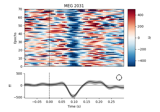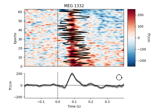# mne.viz.plot_epochs_image¶

mne.viz.plot_epochs_image(epochs, picks=None, sigma=0.0, vmin=None, vmax=None, colorbar=True, order=None, show=True, units=None, scalings=None, cmap=None, fig=None, axes=None, overlay_times=None, combine=None, group_by=None, evoked=True, ts_args=None, title=None, clear=False)[source]

Plot Event Related Potential / Fields image.

Parameters
epochsinstance of Epochs

The epochs.

picks

Channels to include. Slices and lists of integers will be interpreted as channel indices. In lists, channel type strings (e.g., ['meg', 'eeg']) will pick channels of those types, channel name strings (e.g., ['MEG0111', 'MEG2623'] will pick the given channels. Can also be the string values “all” to pick all channels, or “data” to pick data channels. None (default) will pick good data channels. picks interacts with group_by and combine to determine the number of figures generated; see Notes.

sigmafloat

The standard deviation of a Gaussian smoothing window applied along the epochs axis of the image. If 0, no smoothing is applied. Defaults to 0.

vmin

The min value in the image (and the ER[P/F]). The unit is µV for EEG channels, fT for magnetometers and fT/cm for gradiometers. If vmin is None and multiple plots are returned, the limit is equalized within channel types. Hint: to specify the lower limit of the data, use vmin=lambda data: data.min().

vmax

The max value in the image (and the ER[P/F]). The unit is µV for EEG channels, fT for magnetometers and fT/cm for gradiometers. If vmin is None and multiple plots are returned, the limit is equalized within channel types.

colorbarbool

Display or not a colorbar.

order

If not None, order is used to reorder the epochs along the y-axis of the image. If it is an array of int, its length should match the number of good epochs. If it is a callable it should accept two positional parameters (times and data, where data.shape == (len(good_epochs), len(times))) and return an array of indices that will sort data along its first axis.

showbool

Show figure if True.

units

The units of the channel types used for axes labels. If None, defaults to units=dict(eeg='µV', grad='fT/cm', mag='fT').

scalings

The scalings of the channel types to be applied for plotting. If None, defaults to scalings=dict(eeg=1e6, grad=1e13, mag=1e15, eog=1e6).

cmapNone | colormap | (colormap, bool) | ‘interactive’

Colormap. If tuple, the first value indicates the colormap to use and the second value is a boolean defining interactivity. In interactive mode the colors are adjustable by clicking and dragging the colorbar with left and right mouse button. Left mouse button moves the scale up and down and right mouse button adjusts the range. Hitting space bar resets the scale. Up and down arrows can be used to change the colormap. If ‘interactive’, translates to (‘RdBu_r’, True). If None, “RdBu_r” is used, unless the data is all positive, in which case “Reds” is used.

fig

Figure instance to draw the image to. Figure must contain the correct number of axes for drawing the epochs image, the evoked response, and a colorbar (depending on values of evoked and colorbar). If None a new figure is created. Defaults to None.

axes

List of Axes objects in which to draw the image, evoked response, and colorbar (in that order). Length of list must be 1, 2, or 3 (depending on values of colorbar and evoked parameters). If a dict, each entry must be a list of Axes objects with the same constraints as above. If both axes and group_by are dicts, their keys must match. Providing non-None values for both fig and axes results in an error. Defaults to None.

overlay_timesarray_like, shape (n_epochs,) | None

Times (in seconds) at which to draw a line on the corresponding row of the image (e.g., a reaction time associated with each epoch). Note that overlay_times should be ordered to correspond with the Epochs object (i.e., overlay_times corresponds to epochs, etc).

combine

How to combine information across channels. If a str, must be one of ‘mean’, ‘median’, ‘std’ (standard deviation) or ‘gfp’ (global field power). If callable, the callable must accept one positional input (data of shape (n_epochs, n_channels, n_times)) and return an array of shape (n_epochs, n_times). For example:

combine = lambda data: np.median(data, axis=1)


If combine is None, channels are combined by computing GFP, unless group_by is also None and picks is a list of specific channels (not channel types), in which case no combining is performed and each channel gets its own figure. See Notes for further details. Defaults to None.

group_by

Specifies which channels are aggregated into a single figure, with aggregation method determined by the combine parameter. If not None, one Figure is made per dict entry; the dict key will be used as the figure title and the dict values must be lists of picks (either channel names or integer indices of epochs.ch_names). For example:

group_by=dict(Left_ROI=[1, 2, 3, 4], Right_ROI=[5, 6, 7, 8])


Note that within a dict entry all channels must have the same type. group_by interacts with picks and combine to determine the number of figures generated; see Notes. Defaults to None.

evokedbool

Draw the ER[P/F] below the image or not.

ts_args

Arguments passed to a call to plot_compare_evokeds to style the evoked plot below the image. Defaults to an empty dictionary, meaning plot_compare_evokeds will be called with default parameters.

title

If str, will be plotted as figure title. Otherwise, the title will indicate channel(s) or channel type being plotted. Defaults to None.

clearbool

Whether to clear the axes before plotting (if fig or axes are provided). Defaults to False.

Returns
figs

One figure per channel, channel type, or group, depending on values of picks, group_by, and combine. See Notes.

Notes

You can control how channels are aggregated into one figure or plotted in separate figures through a combination of the picks, group_by, and combine parameters. If group_by is a dict, the result is one Figure per dictionary key (for any valid values of picks and combine). If group_by is None, the number and content of the figures generated depends on the values of picks and combine, as summarized in this table:

group_by

picks

combine

result

dict

None, int, list of int, ch_name, list of ch_names, ch_type, list of ch_types

None, string, or callable

1 figure per dict key

None

None, ch_type, list of ch_types

None, string, or callable

1 figure per ch_type

int, ch_name, list of int, list of ch_names

None

1 figure per pick

string or callable

1 figure

## Examples using mne.viz.plot_epochs_image¶